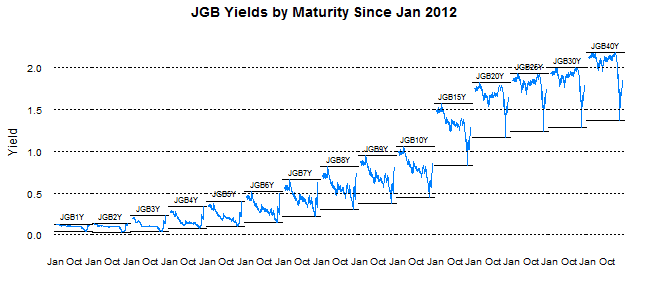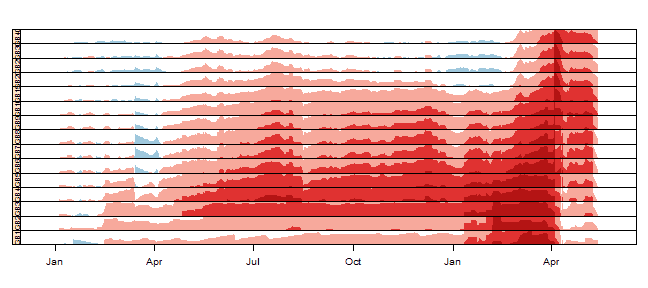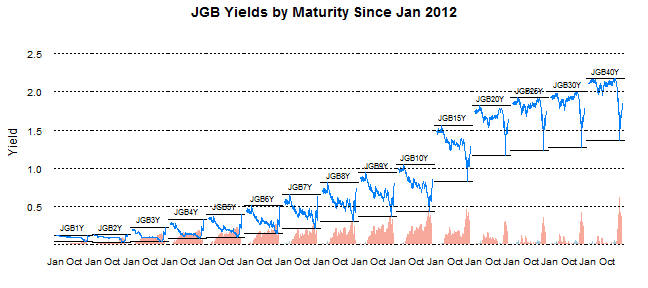Want to share your content on R-bloggers? click here if you have a blog, or here if you don't.

See the last post for all the details. I just could not help creating a couple more.

### Variations on Favorite Plot – Time Series Line of JGB Yields by Maturity

```p2 <- xyplot(value ~ date | indexname, data = jgb.melt,
type = "l", layout = c(length(unique(jgb.melt\$indexname)),
1), panel = function(x, y, ...) {
panel.abline(h = c(min(y), max(y)))
panel.xyplot(x = x, y = y, ...)
panel.text(x = x[length(x)/2], y = max(y),
labels = levels(jgb.melt\$indexname)[panel.number()],
cex = 0.7, pos = 3)
}, scales = list(x = list(tck = c(1, 0), alternating = 1),
y = list(tck = c(1, 0), lwd = c(0, 1))), strip = FALSE,
par.settings = list(axis.line = list(col = 0)),
xlab = NULL, ylab = "Yield", main = "JGB Yields by Maturity Since Jan 2012")
p2 <- p2 + layer(panel.abline(h = pretty(jgb.melt\$value),
lty = 3))
p2
```From TimelyPortfolio

```jgb.xts.diff <- jgb.xts["2012::", ] - matrix(rep(jgb.xts["2012::",
][1, ], NROW(jgb.xts["2012::", ])), ncol = NCOL(jgb.xts),
byrow = TRUE)
jgb.diff.melt <- xtsMelt(jgb.xts.diff)
jgb.diff.melt\$date <- as.Date(jgb.diff.melt\$date)
jgb.diff.melt\$value <- as.numeric(jgb.diff.melt\$value)
jgb.diff.melt\$indexname <- factor(jgb.diff.melt\$indexname,
levels = colnames(jgb.xts))

p4 <- xyplot(value ~ date | indexname, data = jgb.diff.melt,
type = "h")

update(p2, ylim = c(min(jgb.diff.melt\$value), max(jgb.melt\$value) +
0.5)) + p4
```From TimelyPortfolio

```update(p2, ylim = c(min(jgb.diff.melt\$value), max(jgb.melt\$value) +
0.5), par.settings = list(axis.line = list(col = "gray70"))) +
update(p4, panel = function(x, y, col, ...) {
# do color scale from red(negative) to
# blue(positive)
cc.palette <- colorRampPalette(c(brewer.pal("Reds",
n = 9), "white", brewer.pal("Blues",
n = 9)))
cc.levpalette <- cc.palette(20)
cc.levels <- level.colors(y, at = do.breaks(c(-0.3,
0.3), 20), col.regions = cc.levpalette)
panel.xyplot(x = x, y = y, col = cc.levels,
...)
})
```From TimelyPortfolio

```
p5 <- horizonplot(value ~ date | indexname, data = jgb.diff.melt,
layout = c(1, length(unique(jgb.diff.melt\$indexname))),
scales = list(x = list(tck = c(1, 0))), xlab = NULL,
ylab = NULL)

p5
```From TimelyPortfolio

```update(p2, ylim = c(0, max(jgb.melt\$value) + 0.5),
panel = panel.xyplot) + p5 + update(p2, ylim = c(0,
max(jgb.melt\$value)))
```From TimelyPortfolio

### Variations on Yield Curve Evolution with Opacity Color Scale

```# add alpha to colors
addalpha <- function(alpha = 180, cols) {
rgbcomp <- col2rgb(cols)
rgbcomp <- alpha
return(rgb(rgbcomp, rgbcomp, rgbcomp,
rgbcomp, maxColorValue = 255))
}

p3 <- xyplot(value ~ indexname, group = date, data = jgb.melt,
type = "l", lwd = 2, col = sapply(400/(as.numeric(Sys.Date() -
jgb.melt\$date) + 1), FUN = addalpha, cols = brewer.pal("Blues",
n = 9)), main = "JGB Yield Curve Evolution Since Jan 2012")

p3 <- update(asTheEconomist(p3), scales = list(x = list(cex = 0.7))) +
layer(panel.text(x = length(levels(jgb.melt\$indexname)),
y = 0.15, label = "source: Japanese Ministry of Finance",
col = "gray70", font = 3, cex = 0.8, adj = 1))

# make point rather than line
update(p3, type = "p")
```From TimelyPortfolio

```# make point with just most current curve as line
update(p3, type = "p") + xyplot(value ~ indexname,
data = jgb.melt[which(jgb.melt\$date == max(jgb.melt\$date)),
], type = "l", col = brewer.pal("Blues", n = 9))
```From TimelyPortfolio